# How to Calculate and Solve for Mass, Velocity and Kinetic Energy | The Calculator Encyclopedia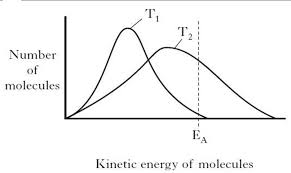The image above represents kinetic energy.

To compute for the kinetic energy, two essential parameters are needed and these parameters are mass (m) and velocity (v).

The formula for calculating the kinetic energy:

K.E. = 0.5mv²

Where;
K.E. = Kinetic Energy
m = Mass
v = Velocity

Let’s solve an example;
Find the kinetic energy when the mass is 6 and the velocity is 18.

This implies that;
m = Mass = 6
v = Velocity = 18

K.E. = 0.5mv²
K.E. = 0.5[6 x 18²]
K.E. = 0.5[6 x 324]
K.E. = 0.5
K.E. = 972

Therefore, the kinetic energy is 972 Joules (J).

Calculating the Mass when Kinetic Energy and Velocity is Given.

m = K.E / 0.5v2

Where;
m = Mass
K.E. = Kinetic Energy
v = Velocity

Let’s solve an example;
Find the mass when the kinetic energy is 320 and a velocity of 20.

This implies that;
K.E. = Kinetic Energy = 320
v = Velocity = 20

m = K.E / 0.5v2
m = 320 / 0.5 x 202
m = 320 / 0.5 x 40
m = 320 / 20
m = 16

Therefore, the mass is 16 kg.

Calculating the Velocity  when Kinetic Energy and Mass is Given.

v = √K.E / 0.5m

Where;
v = Velocity
m = Mass
K.E. = Kinetic Energy

Let’s solve an example;
Find the velocity when the kinetic energy is 120 and a of mass 10.

This implies that;
m = Mass = 10
K.E. = Kinetic Energy = 120

v = √K.E / 0.5m
v = √120 / 0.5 x 10
v = √120 / 5
v = √24
v = 4.89

Therefore, the velocity is 4.89.

Nickzom Calculator – The Calculator Encyclopedia is capable of calculating the kinetic energy.

To get the answer and workings of the kinetic energy using the Nickzom Calculator – The Calculator Encyclopedia. First, you need to obtain the app.

You can get this app via any of these means:

To get access to the professional version via web, you need to register and subscribe for NGN 1,500 per annum to have utter access to all functionalities.
You can also try the demo version via https://www.nickzom.org/calculator

Once, you have obtained the calculator encyclopedia app, proceed to the Calculator Map, then click on Work, Energy and Time under Physics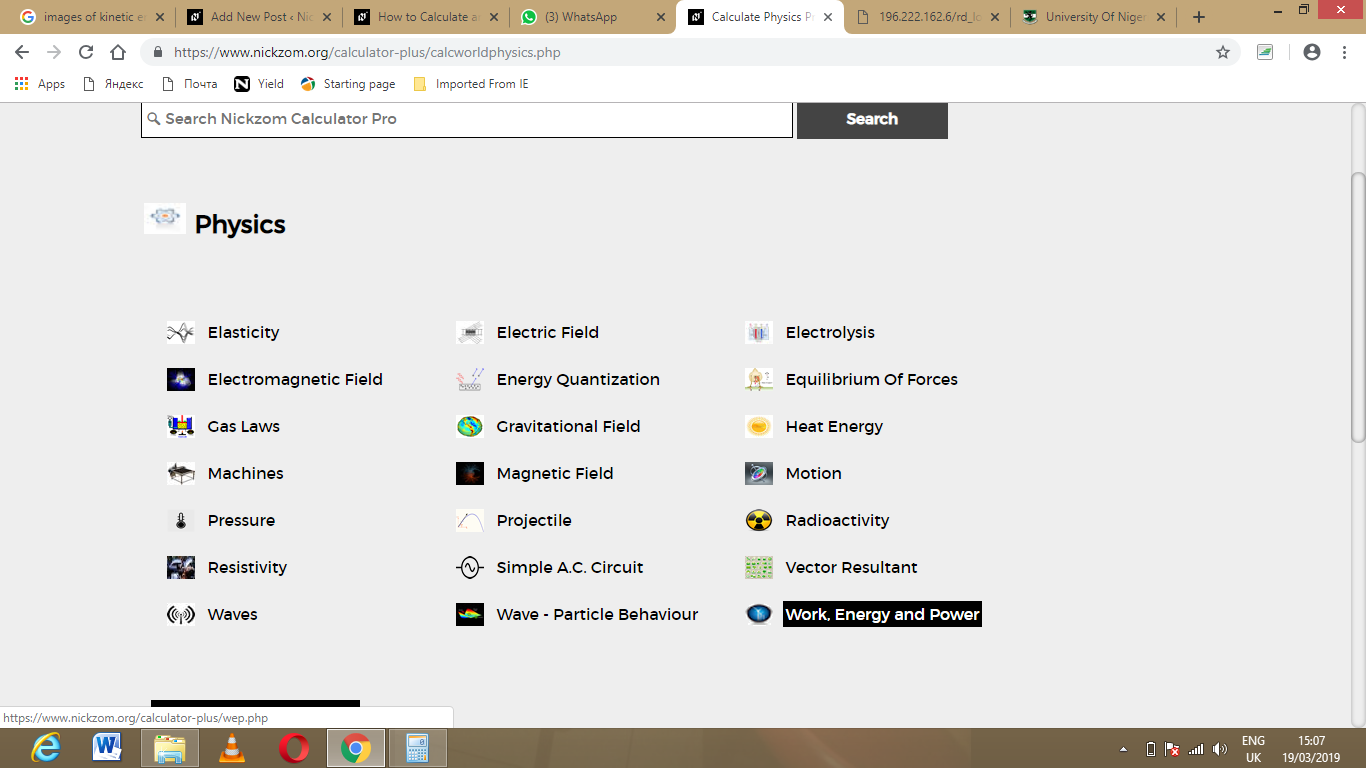Now, Click on Kinetic energy under Work, Energy and TimeThe screenshot below displays the page or activity to enter your values, to get the answer for the kinetic energy according to the respective parameters which are the mass (m) and velocity (v).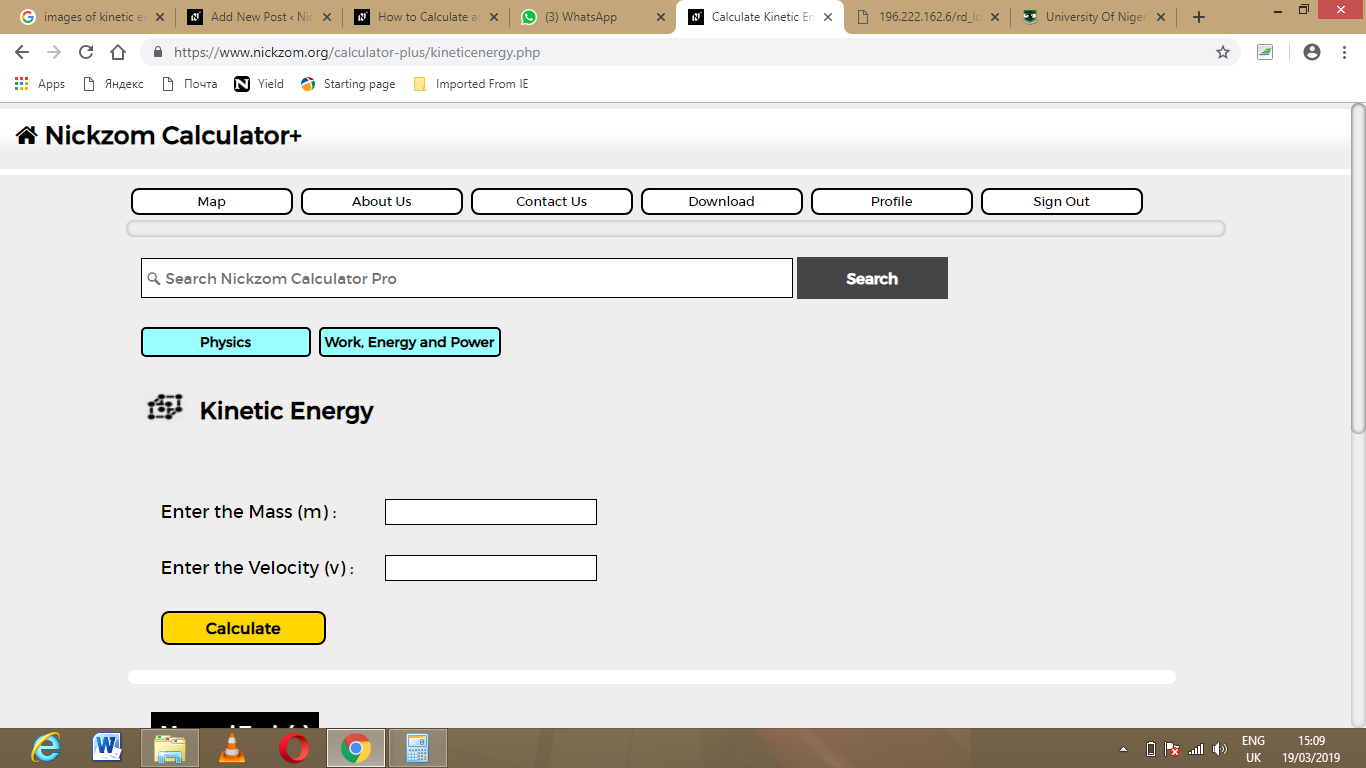Now, enter the values appropriately and accordingly for the parameters as required by the mass (m) is 6 and velocity (v) is 18.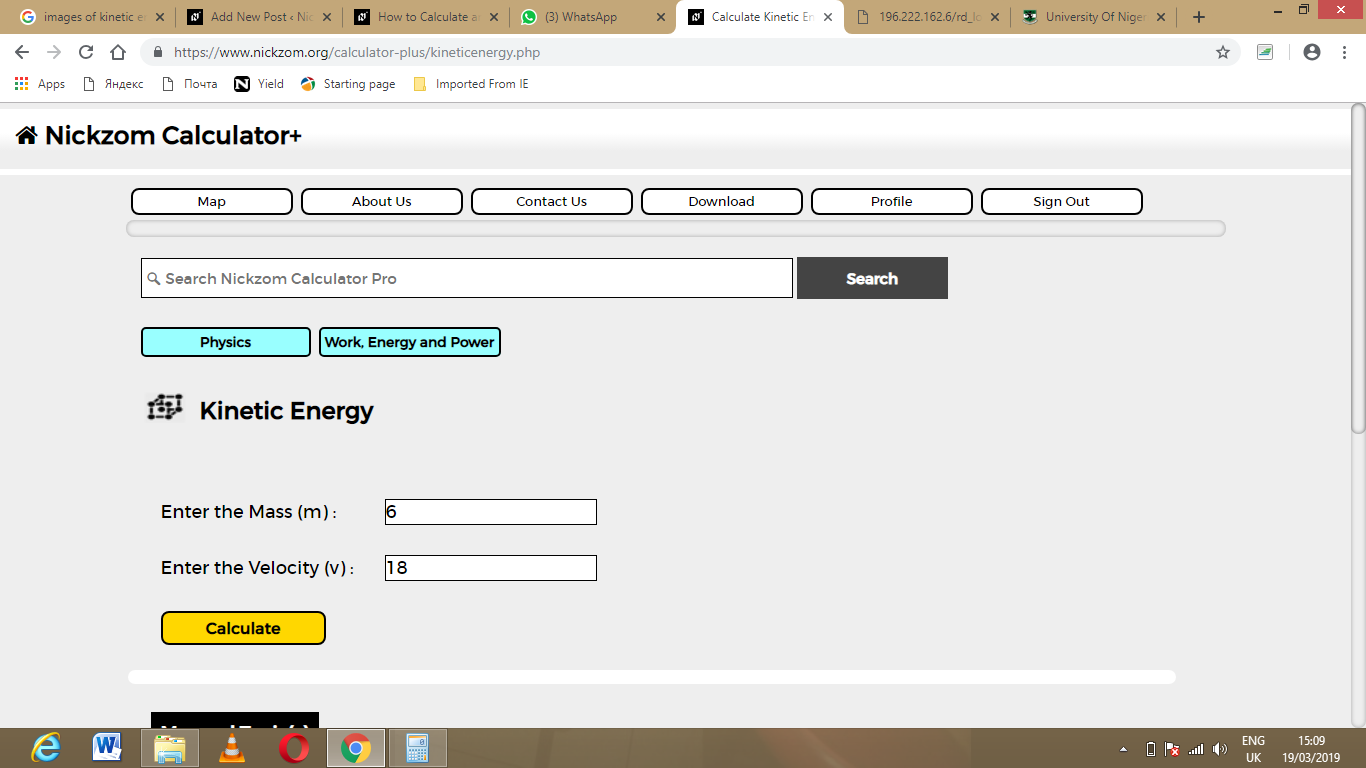Finally, Click on Calculate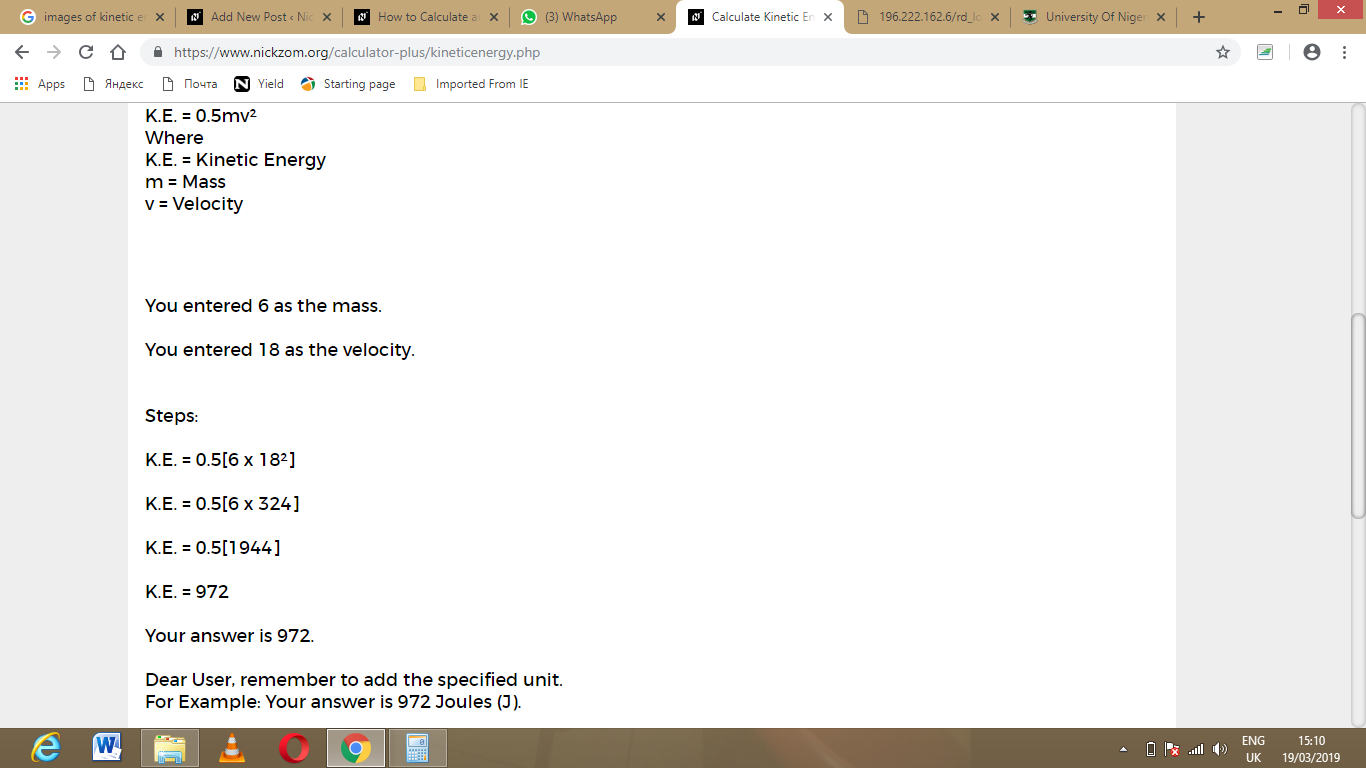As you can see from the screenshot above, Nickzom Calculator – The Calculator Encyclopedia solves for the kinetic energy and presents the formula, workings and steps too.